Lecture 20 - PHY294H

Magnetic dipole

We found that the torque on current loops could be written in the form,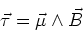(1)

where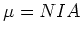for a N-loop coil of area A carrying current I. The magnetic dipole direction is given by the right hand rule with the fingers curling in the direction of the current flow and the thumb giving the direction of the magnetic moment. The torque is zero when the magnetic dipole is aligned or anti-aligned with the magnetic field, while the torque is a maximum when the magnetic dipole is perpendicular to the magnetic field. As in the case of electric dipoles, it is easy to show that the energy of the magnetic dipole is given by,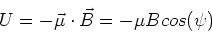(2)

where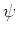is the angle between the direction of the magnetic field and the direction of the magnetic moment. If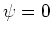the moment and the field are aligned,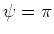when they are anti-aligned.

Example 29.6

A current loop of magnetic moment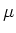in a constant field, B, and moment of inertia about its center, IM. It is displaced by a small anglefrom the aligned state. If the current loop is released at t=0 find the subsequent motion of the loop.

The torque on the loop is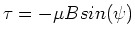. This torque leads to an angular acceleration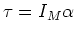. Equating these two expressions, we have,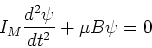(3)

where we have used the small angle approximation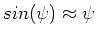. This has solution,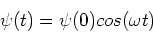(4)

where.

FGT 29.54

In this problem we cannot usebecause the current does not go around the loop. The dipole moment formula only works for current loops. Instead, this is a current divider. The current V1=V2, so I1R1 = I2R2. We also have I=I1+I2, so the currents I1 and I2 are given by,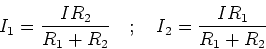(5)

The torque on the loop is then given by,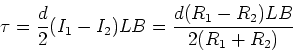(6)